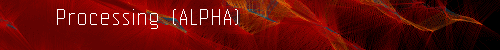FAQ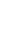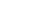CoverThis is the archive Discourse for the Processing (ALPHA) software. Please visit the new Processing forum for current information.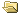Processing 1.0 _ALPHA_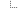Programming Questions & Help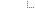Programs (Moderators: fry, REAS)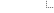fractal brain teaser « Previous topic | Next topic »

 Pages: 1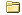Author Topic: fractal brain teaser  (Read 3027 times)
st33d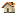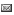fractal brain teaser « on: Apr 5th, 2005, 12:51am »

I've been rolling this around for a week now coming up with different methods but still no success so I'm asking for help.

I intend to draw the serpinski fractal but the only example code I found was for drawing it pixel by pixel (I want to make a vector version down to a certain depth).

I'm sure there's a recursive algorithm for doing it. Here's how far I've got laying out the idea:
Code:
 float Max = 200; void setup(){   size(400,400);   noFill(); } void loop(){   background(255);   translate(200,200);   push();   equilateral(0,0,Max);   rotate(PI);   equilateral(0,0,Max/2);   pop();   serpinski(0,0,Max);   float [] temp = triPush(0,0,Max/2);   serpinski(temp,temp,Max/2);   serpinski(temp,temp,Max/2);   serpinski(temp,temp,Max/2);   float [] temp1 = triPush(temp,temp,Max/4);   float [] temp2 = triPush(temp,temp,Max/4);   float [] temp3 = triPush(temp,temp,Max/4);   for(int i = 0; i < temp1.length/2; i++){   serpinski(temp1[i*2],temp1[(i*2)+1],Max/4);   }   for(int i = 0; i < temp2.length/2; i++){   serpinski(temp2[i*2],temp2[(i*2)+1],Max/4);   }   for(int i = 0; i < temp3.length/2; i++){   serpinski(temp3[i*2],temp3[(i*2)+1],Max/4);   } } //Frederik's triangle: void equilateral(float x, float y, float r){ float [] points = triPush(x,y,r); beginShape(TRIANGLES); vertex(points,points); vertex(points,points); vertex(points,points);   endShape(); } void serpinski(float x,float y,float mag){     float [] temp = triPush(x,y,mag/2);   for (int i = 0; i < temp.length>>1; i++){   push();   translate(temp[i*2],temp[(i*2)+1]);   rotate(PI);   equilateral(0,0,mag/4);   pop();   } } float [] triPush(float Xc, float Yc, float R){ float theta = HALF_PI; float [] temp = new float; temp = Xc+R*cos(theta); temp = Yc+R*sin(theta); temp = Xc+R*cos(theta+TWO_PI/3.0); temp = Yc+R*sin(theta+TWO_PI/3.0); temp = Xc+R*cos(theta+TWO_PI/1.5); temp = Yc+R*sin(theta+TWO_PI/1.5); return temp; }

There's a structure there. I just can't figure out how to compress it. Any help?

I could murder a pint.
kurol

 Re: fractal brain teaser « Reply #1 on: Apr 5th, 2005, 9:34am »

here's what I got so far.

Code:
 float heightoftriangle = cos(radians(30));    // universal constant   int max_depth = 4;   void setup(){   size(400,400);   noFill(); } void draw(){   background(255);     //eqtri(0,0,width);// Main Triangle     serpinski(1); }   // x and y are some coords, b = base or length of side void eqtri(float x, float y, float b){   triangle(x,y,x+b,y,x+b/2,y+b*heightoftriangle); }   void serpinski(int depth){   if (depth <= max_depth) {     float newb = width/pow(2,depth);       //push();     eqtri(0,0,newb);     serpinski(depth+1);     //pop();       push();     translate(newb,0);     eqtri(0,0,newb);     serpinski(depth+1);     pop();       push();     translate(newb/2,newb*heightoftriangle);     eqtri(0,0,newb);     serpinski(depth+1);     pop();   } }

It's not quite right. You should be able to control the depth from the functional call in draw(), but can't. and a depth of 0 should just give you 1 triangle.

and since all the divisions are by powers of 2, how do I optimize that?
 « Last Edit: Apr 5th, 2005, 9:54am by kurol »

I hate programming . . .kurol

 Re: fractal brain teaser « Reply #2 on: Apr 5th, 2005, 10:11am »

And here's my *final* version. This one is different because it can do 0 levels deep, and doesn't draw the triangles until the last moment. I've also learned how to shift bits, and it's interactive, this one is.

Code:
 float heightoftriangle = cos(radians(30));    // universal constant   void setup(){   size(512,512);      // 512 divides so nicely   noFill(); } int serpinski_depth = 3; void loop(){   background(255);   framerate(10);    // should be fast enough   serpinski(0,serpinski_depth); }   void mouseDragged(){ if (mouseX > pmouseX) { serpinski_depth++; } else if (mouseX < pmouseX) { serpinski_depth--; }   serpinski_depth = constrain(serpinski_depth,0,10); }   // b = base or length of side void eqtri(int b){ triangle(0,0,b,0,b>>1,b*heightoftriangle); }   void serpinski(int depth, int max_depth){   if (depth <= max_depth) {     int newb = width>>depth;   // we can avoid rounding errors from excessive scale() use     if (depth == max_depth) { eqtri(newb); }     serpinski(depth+1, max_depth);       if (depth > 0) {  push();  translate(newb,0);     if (depth == max_depth) { eqtri(newb); }  serpinski(depth+1, max_depth);    translate(-newb>>1,newb*heightoftriangle);     if (depth == max_depth) { eqtri(newb); }  serpinski(depth+1, max_depth);  pop();     }   } }

http://www.kurol.net/p5/serpinski/
I left my old code because I think it's more readable.
 « Last Edit: Apr 5th, 2005, 10:41am by kurol »

I hate programming . . .st33dRe: fractal brain teaser « Reply #3 on: Apr 5th, 2005, 10:48pm »

http://www.robotacid.com/misc/serpinski.html

Multiple recursion. I'll admit that's beyond me. I quit math at GCSE level after they refused to let me sit the higher paper.

How the hell do you manage to get those images uploaded to the forum?

I could murder a pint.
kurol

 Re: fractal brain teaser « Reply #4 on: Apr 6th, 2005, 8:28am »

that is pretty cool

most of my images are hosted by imageshack. I have no idea what their business model is. . .

I hate programming . . .Pages: 1

 Forum Jump: ----------------------------- Discussion -----------------------------  - Community, Collaboration, Status   - Events, Publications, Propaganda   - General Processing Discussion ----------------------------- Programming Questions & Help -----------------------------  - Syntax => Programs   - Integration ----------------------------- Topics & Contributions -----------------------------  - Tools   - Responsive Form, Games   - Information Visualization   - Simulation, Artificial Life   - Tangible Computing   - Automated Systems   - Sound   - Video, Camera   - Beyond Categories ----------------------------- Suggestions -----------------------------  - Software Suggestions   - Website, Reference, Example Suggestions ----------------------------- Bugs -----------------------------  - Software Bugs   - Website, Reference, Example Bugs   - Bug Fixes, Implemented Suggestions ----------------------------- Teaching -----------------------------  - Course Blueprints   - Theory and Practice « Previous topic | Next topic »